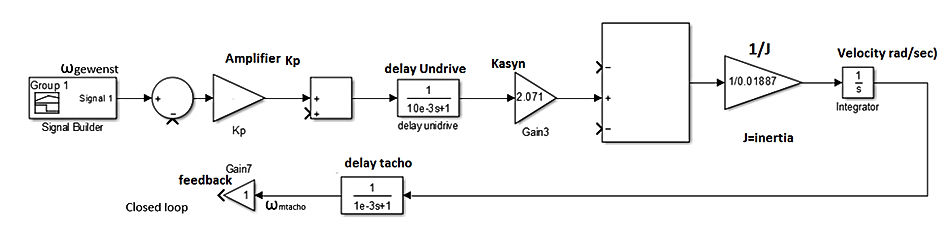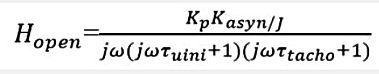naver-site-verification: naver47238a1b6bfbb19a2fd4b619734fa9a6.html
top of page

# The Bode diagram with the Ti-84 Plus (CE) page 1

## Explanation of the Bode diagram program : P-control

#### The Bode diagram is useful for determining the stability of a control system  (phase margin  and  gain margin). It is an important subject in Electrical Engineering. With this Ti-84 Basic program, it is possible to plot the Bode diagram and to determine the proportional gain for a desired phase margin or gain margin. As an example, we treat a speed control system of which we have to determine the proportional gain for a phase margin of 60 degrees. The phase and the gain margin are determined of an open loop system, See the figure below. The example is related to a real existing drive system at Avans University.For Kp=1 the Bode diagram of Hopen is plotted, Kasyn=2.071Nm/A, J=0.018887kgm^2,
tauuni=10msec , tautacho=1msec.

The Bode Program menu has 5 options.

1: New transfer function open loop= Hs

2: The open loop function * Controller = H(s)*Hr

3: existing model is used for adapting the scaling of  the axes

4: Closed loop =(Hs*Hr/(1+Hs*Hr)

5: StopOpen loop transfer function. Green= amplitude, Red=phase/10 (to fit in amplitude screen). @ 0dB the phase=-13.48*10=-134.8 degrees. Which is a phase margin of 180-134.8=45.2 degreesTo achieve 0dB of total gain, the gain Kp should be -5.65 dB =10^(-5.65/20)=0.52. The corresponding frequency equals 51.12 rad/sec.
If a P-control is added by inserting Hr(s)=0.52, and we run the program Bode again, then 0dB gives a phase margin of 180-120-= 60deg phase margin. See figures below. Remember that the real phase has to be multiplied by 10 (12*10=120deg). The corresponding frequency  =  51.12 rad/sec, which = 10^1.71A check with Simulink (Matlab) gives the same result, for Kp=0.52 there is a phase margin of 60.1 degrees at a corresponding radian frequency of 51 rad/sec

bottom of page